##### GED Test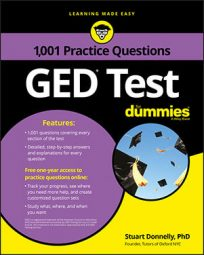Some questions on the GED Math test will take geometry problems to the next level by combining two-dimensional shapes and asking you to calculate their total perimeter or area.

## Practice Questions

Both questions are based on the following image, which shows a rectangle with a semicircular top.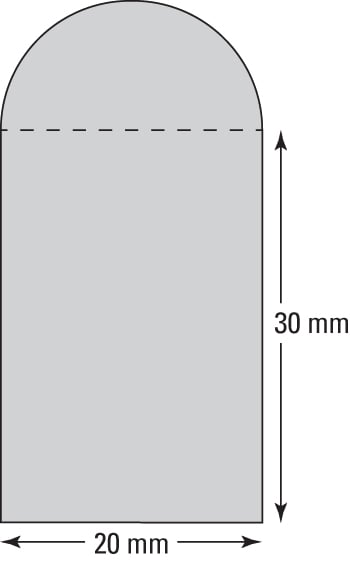1. What is the perimeter of the shape?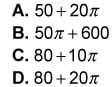2. What is the area of the shape?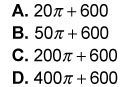1. The correct answer is C.

The length of the curved part of the semicircle equals half the circumference of the circle (whose diameter equals 20 mm). The circumference of a full circle is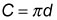so the length is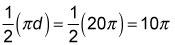The perimeter of the shape consists of this curved length plus the other three sides of the rectangle: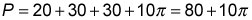The correct answer is Choice (C).

2. The correct answer is B.

The area of the semicircle is half the area of the full circle (whose diameter equals 20 mm and whose radius equals 10 mm). The area of a full circle is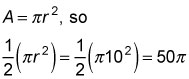The area of the shape consists of the area of the semicircle plus the area of the rectangle: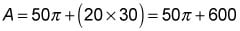Hence, the correct answer is Choice (B).